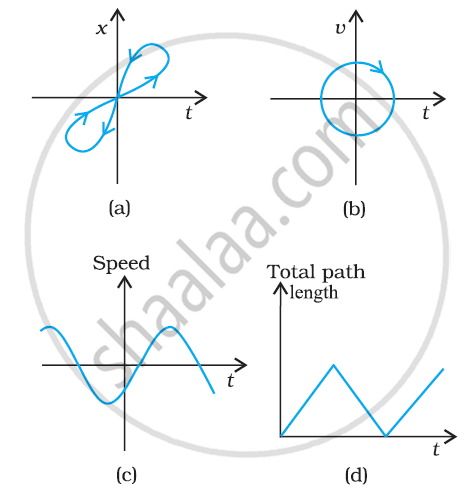CBSE (Science) Class 11CBSE
Share

# Look at the Graphs (A) to (D) Figure Carefully and State, with Reasons, Which of These Cannot Possibly Represent One-dimensional Motion of a Particle - CBSE (Science) Class 11 - Physics

ConceptPosition, Path Length and Displacement

#### Question

Look at the graphs (a) to (d) Figure carefully and state, with reasons, which of these cannot possibly represent one-dimensional motion of a particle.#### Solution 1

(a) The given x-t graph, shown in (a), does not represent one-dimensional motion of the particle. This is because a particle cannot have two positions at the same instant of time.

(b) The given v-t graph, shown in (b), does not represent one-dimensional motion of the particle. This is because a particle can never have two values of velocity at the same instant of time.

(c) The given v-t graph, shown in (c), does not represent one-dimensional motion of the particle. This is because speed being a scalar quantity cannot be negative.

(d) The given v-t graph, shown in (d), does not represent one-dimensional motion of the particle. This is because the total path length travelled by the particle cannot decrease with time.

#### Solution 2

None of the four graph represent a possible one-dimensional motion. In graphs (a) and (b) motions are definitely two dimensional.

Graph (a) represents two positions at the same time which is not possible.

In graph (b) opposite motion is visible at the same time.

The graph (c) is not correct since it shows that the particle has negative speed at a certain instant. Speed is always positive.

In graph (d) path length is shown as increasing as well as decreasing. Path length never decreases

Is there an error in this question or solution?

#### APPEARS IN

NCERT Solution for Physics Textbook for Class 11 (2018 to Current)
Chapter 3: Motion in a Straight Line
Q: 16 | Page no. 57

#### Video TutorialsVIEW ALL 

Solution Look at the Graphs (A) to (D) Figure Carefully and State, with Reasons, Which of These Cannot Possibly Represent One-dimensional Motion of a Particle Concept: Position, Path Length and Displacement.
S